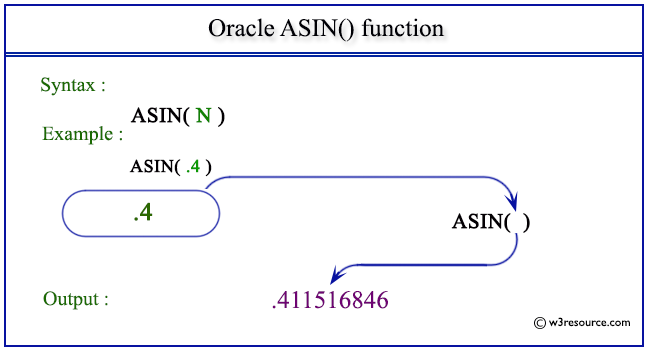# Oracle ASIN() function

## Description

The ASIN() is used to calculate the angle value (in radians) of a specified sine. The function returns a value in the range of -pi/2 to pi/2, expressed in radians and returns an error message when the value of the number is not between the ranges -1 to 1.
The function takes any numeric or nonnumeric data type (can be implicitly converted to a numeric data type) as an argument.
If the argument is BINARY_FLOAT, then the function returns BINARY_DOUBLE. Otherwise, the function returns the same numeric data type as the argument.

Syntax:

`ASIN(N)`

Parameters:

Name Description
N A number whose arch sine value is to be retrieved.

Pictorial Presentation of ASIN() functionExample

The statement below will return an error because the number specified as an argument is exceeding the range which can be accepted.

``````SELECT ASIN(4) FROM dual;
```
```

Here is the result.

```SELECT ASIN(4) FROM dual
*
ERROR at line 1:
ORA-01428: argument '4' is out of range
```

Example: ASIN() function using fractional number

The statement below will return the arc sine value of the number specified as an argument.

``````SELECT ASIN(.4) FROM dual;
```
```

Sample Output:

```  ASIN(.4)
----------
.411516846
```

Previous: ACOS
Next: ATAN

﻿